## Example Questions

← Previous 1 3 4 5 6 7 8 9 21 22

### Example Question #1 : How To Find The Domain Of A Function

What is the domain of the function f(x) = 2/(7x – 1) ?

x < (1/7)

x > (–1/7)

x < (–1/7)

x > (1/7)

x ≠ (1/7)

x ≠ (1/7)

Explanation:

The domain means what real number can you plug in that would still make the function work. For this case, we have to worry about the denominator so that it does not equal 0, so we solve the following. 7x – 1 = 0, 7x = 1, x = 1/7, so when x ≠ 1/7 the function will work.

### Example Question #1 : Algebraic Functions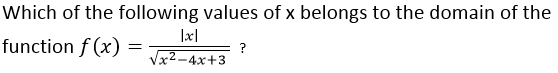x = 2

x = 3

x = 1

x = 0

x = 1.5

x = 0

Explanation: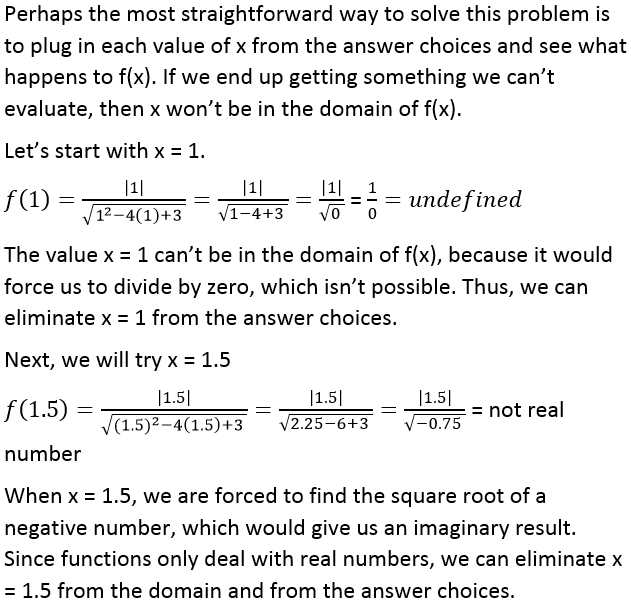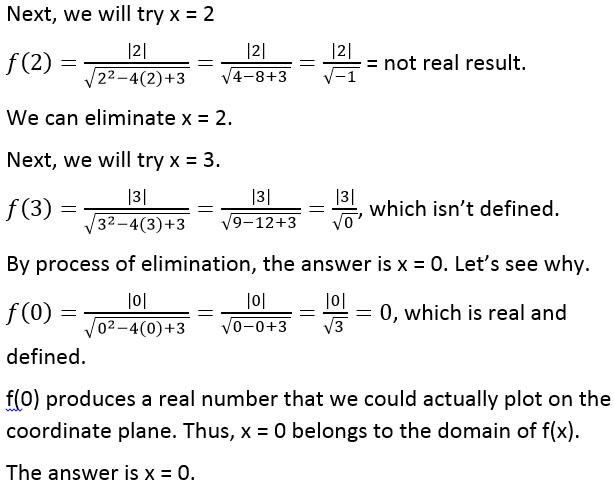### Example Question #2 : How To Find The Domain Of A Function

What is the domain of the set of ordered pairs {(2, –3), (4, 6), (–3, 5), (–2, 5)}?

{–3, 5, 6}

{2, 4}

{–2, –3, 2, 4}

{all real numbers}

{all x-values such that x = 2n + 1 for all n}

{–2, –3, 2, 4}

Explanation:

The domain of a function or relation is the set of all possible x-values. Here that is simply a list of the x-coordinates of all of the coordinate pairs. So the domain is {–2, –3, 2, 4}.

### Example Question #1 : Algebraic Functions

Let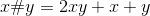.

Ifandare both negative integers larger than negative five, what is the smallest value possible for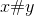?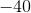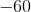Explanation:

Because x and y must be negative integers greater than negative five, then x and y can only be equal to the following values:

x can equal -4, -3, -2, or -1

y can equal -4, -3, -2, or -1

Now we can try all of the combinations of x and y, and see what x # y would equal. It is helpful to note that x # y is the same as y # x because 2yx + y + x = 2xy + x  + y. This means that the order of x and y doesn't matter.

-4 # -4 = 2(-4)(-4) + -4 + -4 = 24

-4 # -3 = 2(-4)(-3) + -4 + -3 = 17

-4 # -2 = 10

-4 # -1 = 3

-3 # -3 = 12

-3 # -2 = 7

-3 # -1 = 2

-2 # -2 = 4

-2 # -1 = 1

-1 # -1 = 0

We don't need to find -3 # -4, -2 # -4, etc, because x # y = y # x .

The smallest value of x # y must be 0.

### Example Question #1 : How To Find The Domain Of A Function

Which of the following functions has a domain that includes all real values of?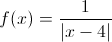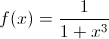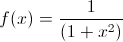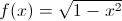Explanation:

The domain of a function includes all of the values of x for which that function is defined. In other words, the domain is all of the real values of x that will produce a real number. Let's look at the domains of each function one at a time.

First, let's examineIn general, when we are examining the domain of a function, we want to find places where we end up with zeros in the denominators or square-roots of negative numbers. For example, in the function, if we let x = 4, then we would be forced to evaluate 1/0, which isn't possible. We can never divide by zero. Thus, this function is not defined over all real values of x. We can eliminate it from the answer choices.

Next, let's look at. Let's set the denominator equal to zero to see if there are any values of x which might give us a zero in the denominator.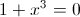Subtract one from both sides.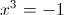Take the cube root of both sides.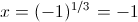Thus, if x = –1, then f(x) will be equal to 1/0, which isn't defined, because we can't divide by zero. Therefore, we can eliminate this answer choice.

Now, let's analyze.

We can never take the square root of a negative number. Thus, if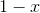, then f(x) won't be defined. For example, if x = 4, then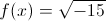, which would produce an imaginary number. Therefore, this function can't be the correct answer.

Next, let's look at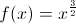. It will help us to rewrite f(x) in a form using square roots. In general,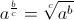. As a result, we can rewrite f(x) as follows: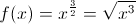. In this form, we can see that if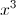is negative, then f(x) won't be defined. Thus, if x = –2, for example, we would be forced to find the square root of –8, which produces an imaginary result. So, this function isn't the correct answer either.

By process of elimination the answer must be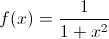. The reasons that this function is defined for all values of x is because the denominator can never be zero. Thus, we can pick any value of x from negative to positive infinity, and we will get a real value for f(x).

The answer is### Example Question #1 : Algebraic Functions

Let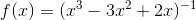. The domain ofincludes which of the following?

I.  1

II.  2

III.  –1

I only

II only

III only

I and II

I, II, and III

III only

Explanation:

The domain of f(x) is defined as all of the values of x for which f(x) is defined.

The first value we have to consider is 1. Let's find f(1):

f(1) = (13 – 3(1)2 +2(1))–1

= (1 – 3(1) + 2)–1

= (1 – 3 + 2)–1

= (0)–1.

Remember that, in general, a–1 = 1/a. Thus, 0–1 = 1/0. However, it is impossible to have 0 in the denominator of a fraction, because it is impossible to divide anything by zero. Thus, 0–1 is undefined. Since f(1) is undefined, 1 cannot belong to the domain of f(x).

Now let's find f(2):

f(2) = (23 – 3(2)2 +2(2))–1

= (8 – 3(4) + 4)–1

=(8 – 12 + 4)–1

= 0–1

Because f(2) is undefined, 2 is not in the domain of f(x).

Finally, we can look at f(–1):

f(–1) = ((–1)3 – 3(–1)2 +2(–1))–1

= (–1 – 3(1) – 2)–1

= (–1 – 3 – 2)–1 = (–6)–1 = –1/6.

f(–1) is defined, so –1 belongs to the domain of f(x).

Therefore only III is in the domain of f(x).

### Example Question #1 : How To Find The Domain Of A Function

What is the domain of the function?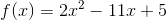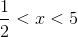All real numbers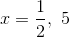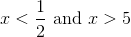All positive integers

All real numbers

Explanation:

The domain of f(x) is all the values of x for which f(x) is defined.

f(x) has no square roots or denominators, so it will always be defined; there are no restrictions on x because any and all values will lead to a real result.

Therefore, the domain is the set of all real numbers.

### Example Question #1 : How To Find The Domain Of A Function

Which of the following represents the domain ofwhere: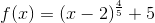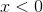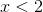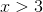is all real numbers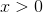is all real numbers

Explanation:

Using our properties of exponents, we could rewriteas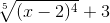This means that when we input, we first subtract 2, then take this to the fourth power, then take the fifth root, and then add three. We want to look at these steps individually and see whether there are any values that wouldn’t work at each step. In other words, we want to know whichvalues we can put into our function at each step without encountering any problems.

The first step is to subtract 2 from. The second step is to take that result and raise it  to the fourth power. We can subtract two from any number, and we can take any number to the fourth power, which means that these steps don't put any restrictions on.

Then we must take the fifth root of a value. The trick to this problem is recognizing that we can take the fifth root of any number, positive or negative, because the function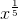is defined for any value of; thus the fact thathas a fifth root in it doesn't put any restrictions on, because we can add three to any number; therefore, the domain foris all real values of.

### Example Question #1 : How To Find The Domain Of A Function

What is the domain of the given function?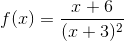x ≠ –3

All real numbers

x ≠ 0

x ≠ 3, –3

x ≠ 3

x ≠ –3

Explanation:

The domain of the function is all real numbers except x = –3. When = –3, f(–3) is undefined.

### Example Question #1 : How To Find The Domain Of A Function

Find the domain of the given function: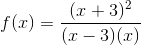All real numbers x such that x ≠ 3

All real numbers x such that x ≠ 1, 0

All real numbers x such that x ≠ 3, 0

All real numbers

All real numbers x such that x ≠ 0

All real numbers x such that x ≠ 3, 0

Explanation:

When = 0 or = 3, the function is undefined due to its denominator.

Thus the domain is all real numbers x, such that x is not equal to 0 or 3.

← Previous 1 3 4 5 6 7 8 9 21 22

### All SAT Math Resources